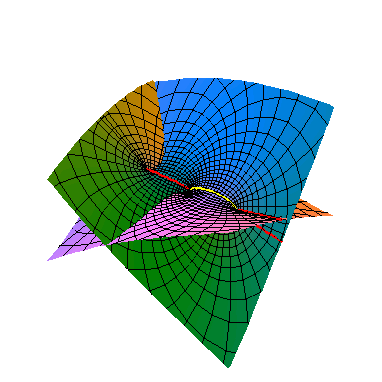This is part of a projection of an elliptic curve to R3.
Click on the graph to find out more.

My preprints or postprints:

1. Geometry of cohomology support loci for local systems I (published in J. Alg. Geom. 1997)
2. Geometry of cohomology support loci II: integrability of Hitchin's map (1997)
3. (with M. Nori) Solvable fundamental groups of algebraic varieties and Kaehler manifolds (published in Compositio Math. 1999)
4. Building mixed Hodge structures (in: The arithmetic and geometry of algebraic cycles, AMS 2000 )
5. (with P. Sastry) Intermediate Jacobians and Hodge Structures of Moduli Spaces (published in Proc. Ind. Acad. Sci. 2000)
6. A class of sheaves satisfying Kodaira's vanishing theorem (published in Math. Ann. 2000)
7. (with S. Archava) Kodaira dimension of symmetric powers ( published in Proc. AMS 2003)
8. Hodge cycles on some moduli spaces
9. (+ appendix by D. Keeler) Frobenius amplitude and strong vanishing theorems for vector bundles (published Duke Math. J, 2004)
10. The Leray spectral sequence is motivic (published in Inventiones Math. 2005)
11. Kahler Solvmanifolds (published Internat. Math. Res. Notices, 2004)
12. Partial regularity and amplitude (published in Amer. J. Math, 2006)
13. (with S-J. Kang) Functoriality of the coniveau filtration Canad. Math. Bull. (2007)
14. Motivation for Hodge cycles (published in Advances in Math, 2006)
15. The Hodge conjecture for rationally connected fivefolds
16. (with S-J. Kang) Coniveau and the Grothendieck group of varieties (published in Mich. Math. J., 2006)
17. (with A. Dhillon) The motive of the moduli stack of G-bundles over the universal curve ( Proc. Ind. Acad. Sci., 2008)
18. Mixed Hodge structures associated to geometric variations (in Cycles, Motives, Shimura Varieties, TIFR Mumbai)
19. Varieties with very little transcendental cohomology (published in Motives and Algebraic Cycles, Fields Inst. 2009)
20. An abelian category of motivic sheaves (Advances in Math 2013)
21. Frobenius Amplitude and Vanishing on Singular Spaces ( Illinois J. Math 2011)
22. (with M. Kumar) Beilinson-Hodge cycles on semiabelian varieties (Math. Res. Let., 2009)
23. The Hodge theoretic fundamental group and its cohomology (published in Geom. of Alg. Cycles, Clay Inst. 2010)
24. (with P. Baktary, J. Wlodarczyk) The combinatorial part of the cohomology of a singular variety.
25. Homomorphisms between Kaehler groups (published in topology of algebraic varieties and singularities, AMS 2011)
26. (with P. Baktary, J. Wlodarczyk) Weights on cohomology, invariants of singularities, and dual complexes (replaces earlier paper; published in Math. Ann.)
27. Hodge theory of cyclic covers branched over a union of hyperplanes (Can J. Math 2014)
28. (with X. Chen, S-J. Kang) The smooth center of the cohomology of a singular variety (published in Hodge theory, complex geometry, and representation theory, AMS 2014)
29. Decomposition of direct images of logarithmic differentials (Internat. Math. Res. Notices 2015)
30. Restrictions on the prime-to-p fundamental group of a smooth projective variety (published in Compositio 2015)
31. Geometric Hodge structures with prescribed Hodge numbers (Recent Advances in Hodge Theory, Cambridge)
32. (with P. Solapurkar) A new class of surfaces with maximal Picard number.
33. (with A. Dimca, R. Hain) On the fundamental groups of normal varieties ( Com. Contemp Math 2016)
34. Toward the structure of fibered fundamental groups of projective varieties. (Journ. de l'Ecole Polytech. 2017)
35. A Lefschetz (1,1) theorem for singular varieties.
36. Simpson's construction of varieties with many local systems. (Hodge theory and L2 analysis)
37. Kodaira-Saito vanishing via Higgs bundles in positive characteristic. (Crelle's Journ. 2019)
38. (with J. Katz, D. B. McReynolds, P. Solapurkar) Integral Gassman equivalence of algebraic and hyperbolic manifolds (Math. Zeit. 2019)
39. Lefschetz decompositions for eigenforms on a Kahler manifold. (Proc. AMS 2018)
40. (with F. Hao, H. Li) Vanishing theorems for parabolic Higgs bundles (Math. Res. Let. 2019)
41. Algebraic cycles on genus two modular fourfolds. (Algebra & Numb. Theory 2019)
42. Motivic sheaves revisited. (to appear in J. Pure & App. Algebra)
43. When are braid groups of manifolds Kahler
44. Hodge cycles and the Leray filtration (to appear in Pacific J. Math)
45. (with B. Wang) Perverse sheaves on varieties with large fundamental groups
46. Residues of connections and the Chevalley-Weil formula for curves (to appear in L'Enseignement Math)
are available from the algebraic geometry archive or go to Google Scholar. Additional preprints/talks
are online in dvi/ps/pdf format. My (somewhat dated) survey article on fundamental groups of smooth projective varieties is contained in the book: Current topics in complex algebraic geometry which is also available electronically at MSRI .

Expository stuff: